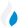•Trade Pi
•Trade
•Exchange
•Trade Pi
•Trade
•Exchange
\$1.1T
Total marketcap
\$65.86B
Total volume
39.99%
BTC dominance
•Trade Pi
•Trade
•Exchange

# LABS to WETH Exchange Rate - 1 LABS Group in WETH

0.00
• ETH 0.00000034
Vol [24h]
\$8,717.99

## labs to weth converter

Exchange Pair Price 24h volume
Uniswap (v2) LABS/WETH \$0.0005642 \$8.73K

## LABS/WETH Exchange Rate Overview

Name Ticker Price % 24h 24h high 24h low 24h volume
LABS Group labs \$0.000587 -4.3388% \$0.0006209 \$0.0005824 \$196.2K
WETH weth \$1,681.31 1.0257% \$1,690.64 \$1,648.74 \$701.26M

Selling 1 LABS you get 0.00 WETH weth.

LABS Group Mar 27, 2021 had the highest price, at that time trading at its all-time high of \$0.1424.

681 days have passed since then, and now the price is 0.40% of the maximum.

Based on the table data, the LABS vs WETH exchange volume is \$8,717.99.

Using the calculator/converter on this page, you can make the necessary calculations with a pair of LABS Group/WETH.

## Q&A

### What is the current LABS to WETH exchange rate?

Right now, the LABS/WETH exchange rate is 0.00.

### What has been the LABS Group to WETH trading volume in the last 24 hours?

Relying on the table data, the LABS Group to WETH exchange volume is \$8,717.99.

### How can I calculate the amount of WETH? / How do I convert my LABS Group to WETH?

You can calculate/convert WETH from LABS Group to WETH converter. Also, you can select other currencies from the drop-down list.

## LABS to WETH Сonversion Table

LABS WETH
900 LABS = 0.0003 WETH
2000 LABS = 0.0006 WETH
4000 LABS = 0.001 WETH
6000 LABS = 0.002 WETH
9000 LABS = 0.003 WETH
20000 LABS = 0.006 WETH
30000 LABS = 0.01 WETH
90000 LABS = 0.03 WETH
200000 LABS = 0.06 WETH
2000000 LABS = 0.6 WETH
20000000 LABS = 6.9 WETH
200000000 LABS = 69.1 WETH
2000000000 LABS = 691.9 WETH